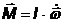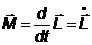# What is rigid body movement

• In contrast to a mass point, the points of application of forces must also be considered with a rigid body. - The Torque is defined as force x force arm: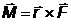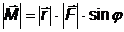The following applies to the direction of the torque: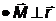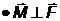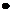Right-hand rule / right-hand screw rule (see sketch)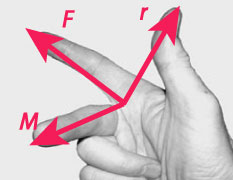The direction of the angular velocity results from the direction of rotation according to the "right-hand screw rule" (see sketch).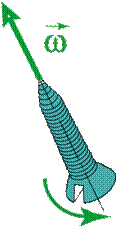• Mass moments of inertia

The mass moment of inertia I. depends on the mass and the body shape (distribution of the mass with respect to the axis of rotation).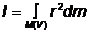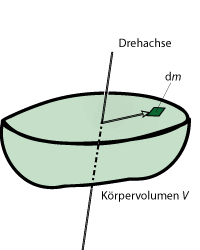• Steiner's theorem

The moment of inertia of a body when rotating about any axis B is related to the moment of inertia with respect to the parallel axis A through the center of gravity in the following relationship: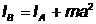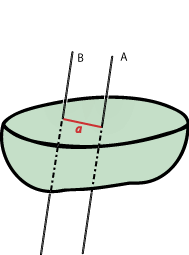• For the Rotational energy of a body: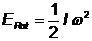• The Angular momentum is defined as: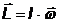• Torque and angular momentum change: A torque leads to an angular acceleration or a change in angular momentum: